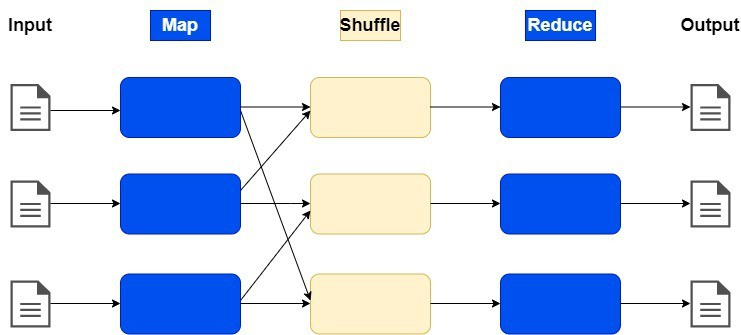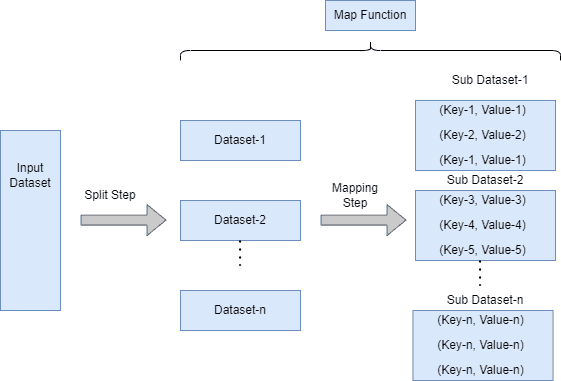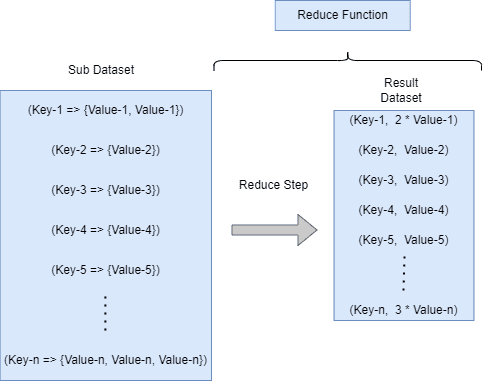## 1. Introduction

In this tutorial, we’re going to present the MapReduce algorithm, a widely adopted programming model of the Apache Hadoop open-source software framework, which was originally developed by Google for determining the rank of web pages via the PageRank algorithm.

MapReduce is capable of expressing distributed computations on large data with a parallel distributed algorithm using a large number of processing nodes. Each job is associated with two sets of tasks, the Map and the Reduce, which are mainly used for querying and selecting data in the Hadoop Distributed File System (HDFS).

## 2. How Does MapReduce Work?

First of all, key-value pairs form the basic data structure in MapReduce. The algorithm receives a set of input key/value pairs and produces a set of key-value pairs as an output. In MapReduce, the designer develops a mapper and a reducer with the following two phases:The order of operations: Map|Shuffle|Reduce

### 2.1. Map Phase

The first phase of MapReduce takes an input pair and produces a set of intermediate key-value pairs (key_1, value_1)[(k_2, v_2)]. The MapReduce library groups together all intermediate values associated with the same intermediate keyand passes them to the reduce function:### 2.2. Reduce Phase

The second phase of MapReduce receives an intermediate keyand a set of values for that key as an input and reduces the data to a simplified form (key_2, [value_2])[(k_3, v_3)]. It combines and merges these values to form a possibly smaller set of values and performs a reduce operation. An iterator is supplied to the reduce function corresponding to the in-between values:When the reduce phase is completed successfully, the results are sent to the Hadoop server.

A MapReduce program includes code for mappers and reducers along with configuration parameters (such as the location and storage information of the input). The developer submits the job to a node of a cluster, and the execution framework handles the rest.

Specific jobs of MapReduce include:

1. Scheduling: The task of dividing jobs into smaller units and coordinating different tasks of different jobs
2. Data & code co-location: In order to achieve data locality, the scheduler starts tasks on the node that holds a particular block of data needed by the task
3. Synchronization: Shuffle and Sort processes form the phase between Map and Reduce, where the output of the Map function is sorted and shuffled based on its key
4. Error & fault handling: If there is no response from a node, the work is assigned to another one to be carried out

## 3. Why Use MapReduce?

### 3.1. Advantages of the Algorithm

Typical database servers face too many bottlenecks and struggle when dealing with massive amounts of data. Also, several data-intensive works suffer from bottlenecks caused by the separation of compute and storage nodes, even if the work that needs to be done is not very processor-demanding. This problem is overcome by using the MapReduce algorithm, which assigns tasks to different nodes and handles every job independently.

In addition,  the distributed file system improves the performance of the executions as it is responsible for organizing the computations so that data is processed sequentially on different nodes and avoiding random data accesses.

### 3.2. Where Is MapReduce Used?

Typical problems/examples that involve the MapReduce algorithm are:

• Distributed grep: The map task produces a line if it fits a specific pattern, while the reduce task formats an identity function that simply reproduces the intermediate data as an output
• Count of URL Access Frequency: The map function processes history file requests of web pages and returns a <URL, N> pair for N requests that will be detected in the corresponding file. The reduce function summarizes all the results for the same URL, thus giving Pairs <URLs, total requests>
• Reverse Web-Link Graph: The map job returns pairs <target, starting point> for each link to one “target” address located on a page called “starting point”. The reduce function joins the list of all “starting points” to a specific one “target,” returning the pairs <target, starting list>
• Inverted Index: The map function reads each document and returns a list of pairs <word, document>, while the reduce function acquires all pairs for a specific word, sorts the documents, and returns a pair <word, list of documents>. A reverse index is formed by the set of all the created pairs

## 4. Example of MapReduce

We consider the problem of counting the number of occurrences of each word in a large collection of documents. The pseudo-code of this problem:Initially, the mapper produces a key-value <docid, doc> pair for every word. Every word works as the key, and the integer works as the value frequency. Then, the reducer sums up all counts that are associated with every single word and creates the desirable key pair.

## 5. Conclusion

In this article we introduced MapReduce, a widely used algorithm due to its capability of handling big data effectively and achieving high levels of parallelism in cluster environments. The ability to process terabytes of data concurrently and accelerate certain algorithms has led this algorithm to gain strong popularity and be widely applied in companies that handle large and complex data.